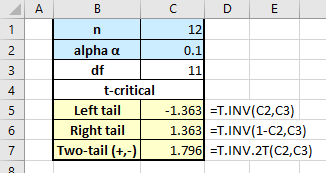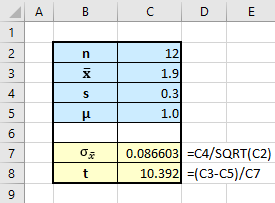# Single-sample t-test & Confidence Interval – Excel & StatCrunch

When we do not know the population standard deviation sigma, σ, and the sample size, n, is less than 30, we use the t-test to evaluate a claim. Consider the following problem:

A scientist thinks the mean waste recycled by adults in the US is now more than one pound per person per day. In a random sample of 12 US adults, the mean waste recycled per day per person is 1.9 pounds with a standard deviation of 0.3 pounds. At a 10% significance level, does the sample data support the claim?

In statistics, it is always a good idea to sketch the situation described in the problem:The sample mean is far to the right of the assumed population mean, µ = 1.

To solve this problem, we start by stating the null and alternative hypotheses:

• The claim includes the phrase “more than” which indicates the math operator is  > .
• Because the null must be a form of equality [ ≤, =, or ≥ ], the claim is the alternative.
•  Ho: µ ≤ 1; Ha: µ > 1.

We can perform the hypothesis test one of two ways:

• Find the critical value of t and determine if the test statistic is in the rejection region.
• Calculate the p-value for the test statistic and compare it to alpha.

Rejection region approach.

• The math operator in the alternative, >, points to the right, so this is a right-tail test.
• To find the critical value of t, we need the degrees of freedom.
• N = 12
• df = n-1 = 11
• Using Excel,• Using the StatCrunch command sequence, Stat > Calculators > T. Enter the df and alpha. Select the “≥” operator to get the right tail. Click Compute. I like StatCrunch for this because it always creates the sketch which helps prevent “dumb” tail errors.The critical value of t is 1.36 and the rejection region is t > 1.36, the area in red.

• Now we need to find the test statistic.Although I can do this using the Compute function in StatCrunch, I like to use Excel for this because I can save the file and reuse on similar problems:Since t = 10.39 falls in the rejection region to the right of t-critical = 1.36, we reject the null hypothesis.

Now let’s use the p-value approach.

• First, using Excel:• Now using StatCrunch: Use the command sequence Stat > T Stats > One Sample > With Summary. Enter the sample mean, sample standard deviation, and n. Note: do not convert the sample s to the standard error (sigma sub x-bar) as we did using Excel because StatCrunch has that step built-in.StatCrunch gives includes the test statistic, 10.392, in the output along with the p-value <0.0001. Again, the decision is to reject the null and conclude:

There is sufficient evidence to support the claim that the mean amount of waste recycled per person per day by US adults is greater than 1 pound.

Confidence Interval for sample mean

Remember, if you are asked for the confidence interval around the sample mean you can get it quickly using the same StatCrunch tool. Click on the Options button in the upper left of the Output box, then Edit, and the dialog box will open. Then just click the radio button next to Confidence interval for µ and click Compute!Getting the confidence interval using Excel is not difficult though you need to recall we use the critical value of t for alpha/2, which is the two-tail critical value even though we were running a one-tail hypothesis test. That trips up a lot of students who just use the t-critical for the rejection area.This site uses Akismet to reduce spam. Learn how your comment data is processed.